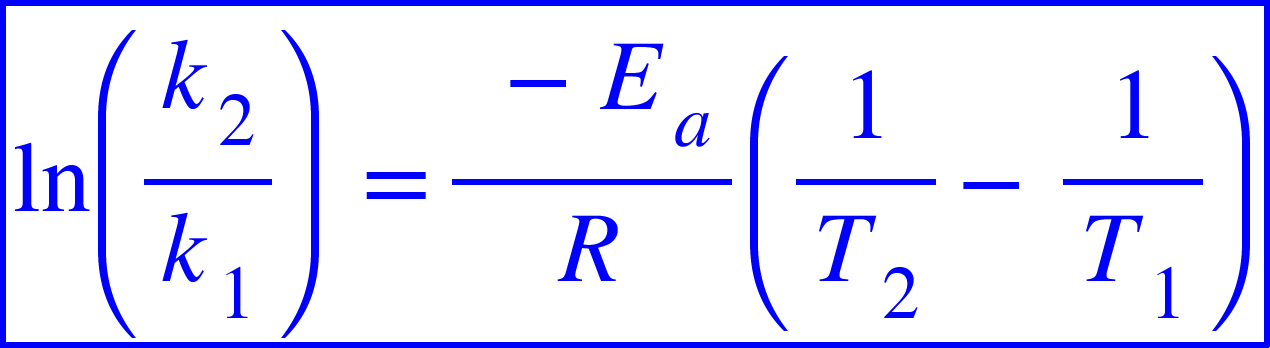# Problem: A first-order reaction has rate constants of 4.6 x 10 -2 s-1 and 8.1 x 10-2 s-1 at 0°C and 20.°C, respectively. What is the value of the activation energy?

###### FREE Expert Solution

The rate constant of a chemical reaction is a temperature dependent quantity.

The relation between the rate constant and temperature is quantified by the Arrhenius equation.We have the following data:

k1 = 4.6x10-2 s-1 at T1 = 0 oC

k2 = 8.1x10-2 s-1 20 °C

R = 8.314 J/mol/K

Ea = ?###### Problem Details

A first-order reaction has rate constants of 4.6 x 10 -2 s-1 and 8.1 x 10-2 s-1 at 0°C and 20.°C, respectively. What is the value of the activation energy?